# Difference between revisions of "Hard disk model"

Hard disks are hard spheres in two dimensions. The hard disk intermolecular pair potential is given by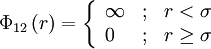$\Phi_{12}\left( r \right) = \left\{ \begin{array}{lll} \infty & ; & r < \sigma \\ 0 & ; & r \ge \sigma \end{array} \right.$

where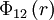$\Phi_{12}\left(r \right)$ is the intermolecular pair potential between two disks at a distance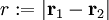$r := |\mathbf{r}_1 - \mathbf{r}_2|$, and$\sigma$ is the diameter of the disk. This page treats hard disks in a two-dimensional space, for three dimensions see the page hard disks in a three dimensional space.

## Phase transitions

Despite the apparent simplicity of this model/system, the phase behaviour and the nature of the phase transitions remains an area of active study ever since the early work of Alder and Wainwright . In a recent publication by Mak  using over 4 million particles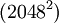$(2048^2)$ one appears to have the phase diagram isotropic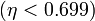$(\eta < 0.699)$, a hexatic phase, and a solid phase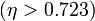$(\eta > 0.723)$ (the maximum possible packing fraction is given by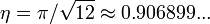$\eta = \pi / \sqrt{12} \approx 0.906899...$ ) . Similar results have been found using the BBGKY hierarchy  and by studying tessellations (the hexatic region: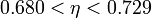$0.680 < \eta < 0.729$) . Also studied via integral equations . Experimental results .

## Equations of state

Main article: Equations of state for hard disks

## Virial coefficients

Main article: Hard sphere: virial coefficients